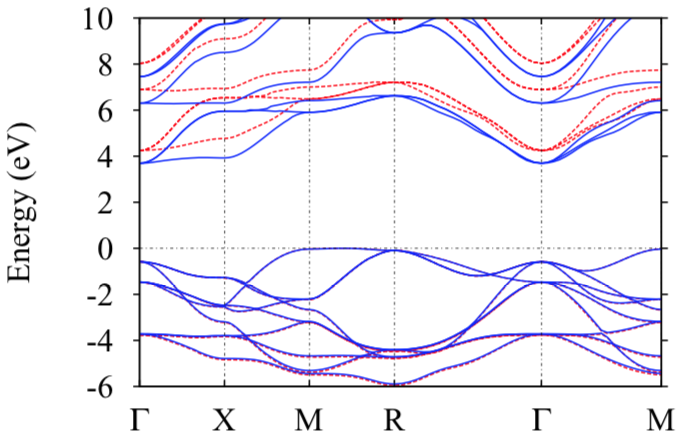news

Frolich contribution to energy band shifts in SrTiO3

The Lambrecht group at Case Western University estimated how phonons modify the band structure in SrTiO3. Isolating the Frolich part of the electron-phonon interaction (which is the dominant contribution for highly polar compounds), they estimated the reduction in the screened coulomb interaction W, and its effect on the QSGW band structure.

SrTiO3 is an insulator, with a bandgap about 1 eV larger than experiment when computed by QSGW. While corresponding LDA-based GW calculations yield bandgaps in much better agreement with experiment, it is a fortutious accident of the LDA starting point. The dielectric function $\varepsilon(\mathbf{q},\omega)$ is overestimated because the LDA bandgap is too small. This error substitutes for other contributions to the screening missing in the RPA. This is immediately evident by comparing the electronic part of the static dielectric function, $\varepsilon_\infty$ to experiment. The LDA slightly overestimes $\varepsilon_\infty$, QSGW underestimates in a very systematic way, by 20%.Both ladder diagrams and the electron-phonon interaction increase the screening, which reduces the bandgap. Contributions from the latter to $W$ can be large at frequencies below the optical phonon mode in ionic compounds, because the lattice contribution to the $\varepsilon(\mathbf{q},\omega)$ can be very large. At small small q the fulll static dielectric constant can be much larger than the electronic part, i.e. $\varepsilon_0/\varepsilon_\infty$ can be very large.

This effect of phonons on bandgaps in polar compounds was first studied by Frolich. This work reconsiders the Frolich term for SrTiO3 in the QSGW context. Even though it is important for microwave frequencies and below, it was found to reduce the gap by about 0.5 eV. The figure compares QSGW bands with and without the Frolich contribution (blue and red bands, respectively).Missing ladder diagrams are believed to account for most of the remainder of the overestimate.

This work was published in Physical Review Materials.

ARTICLE · QSGW · PHONONS